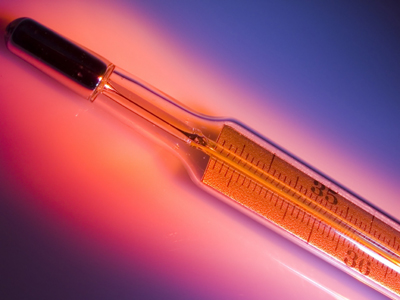A thermometer can be used for measuring temperature.

# Measurement - The Metric System - Temperature (Fahrenheit to Celsius)

This Math quiz is called 'Measurement - The Metric System - Temperature (Fahrenheit to Celsius)' and it has been written by teachers to help you if you are studying the subject at middle school. Playing educational quizzes is a fabulous way to learn if you are in the 6th, 7th or 8th grade - aged 11 to 14.

It costs only \$12.50 per month to play this quiz and over 3,500 others that help you with your school work. You can subscribe on the page at Join Us

The metric system is the mathematical system used in almost every country of the world. In fact, only three countries do not use it and those are the United States, Liberia and Burma.

The metric system is a much easier system as it deals in conversions of base 10. However, not only are measurements of length/distance, weight/mass and volume done in the metric system but so is the measurement of temperature.

In the United States, the measurement of temperature is done in Fahrenheit such as 85 degrees Fahrenheit or 85º F. In 1714 a German physicist, Gabriel Daniel Fahrenheit, invented the mercury thermometer with a scale to record temperatures and that scale bears his name.

However, just as most countries use the metric system over the American system or English system, many countries use Celsius over Fahrenheit to measure temperature. And where did the Celsius measurement come from? Well, in 1742, Anders Celsius, a Swedish astronomer, devised the centigrade thermometer which now bears his name.

Whether you go by Fahrenheit or Celsius, it really is a good idea to be familiar with both because you will encounter both throughout your life and throughout the world. So, in order to understand how they relate, we need to convert one to the other. You’ll be amazed at just how simple it really is to do this.

So let’s see how we convert temperatures from Fahrenheit to Celsius. You will need to remember the formula as shown below.

Go straight to Quiz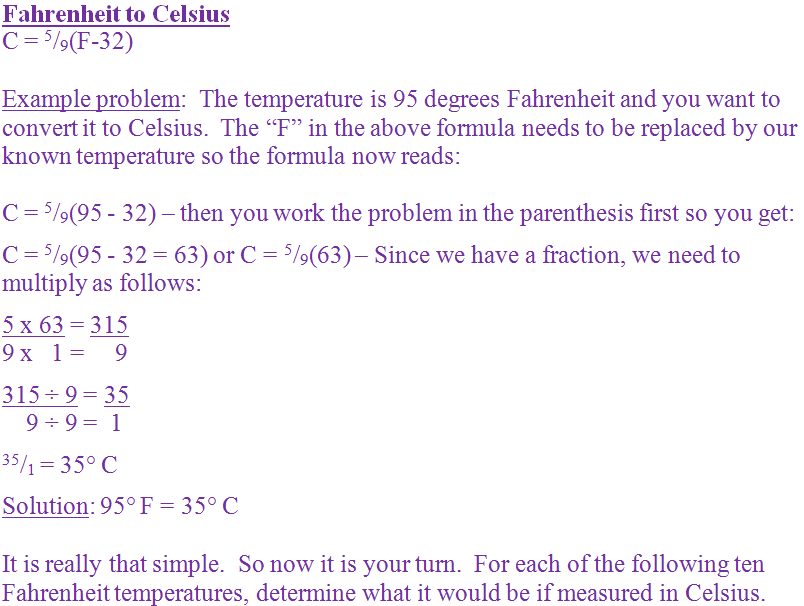Question 1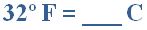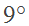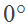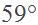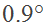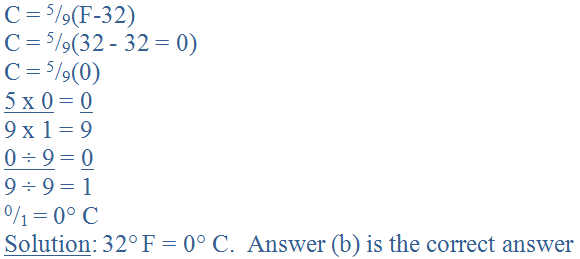Question 2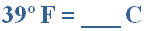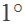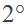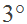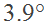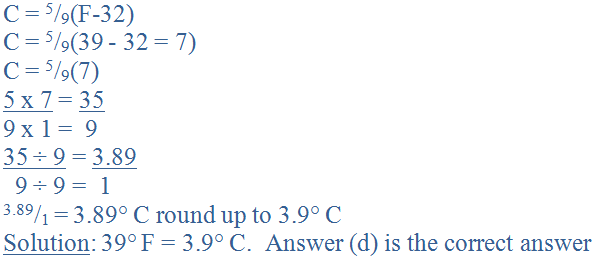Question 3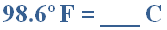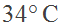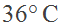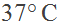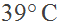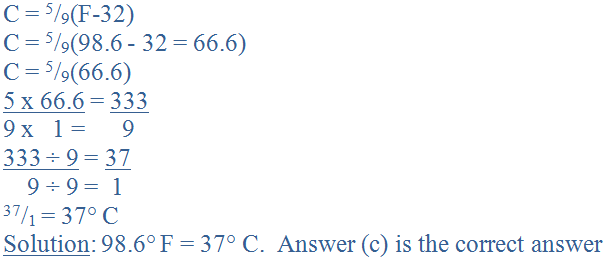Question 4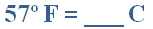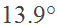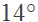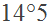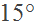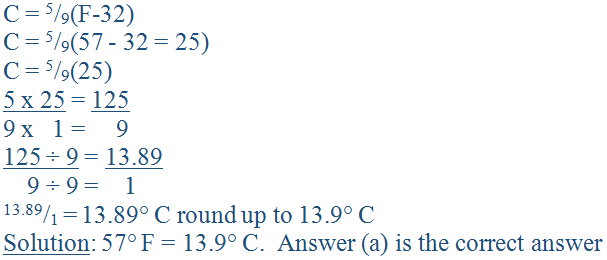Question 5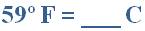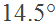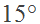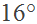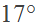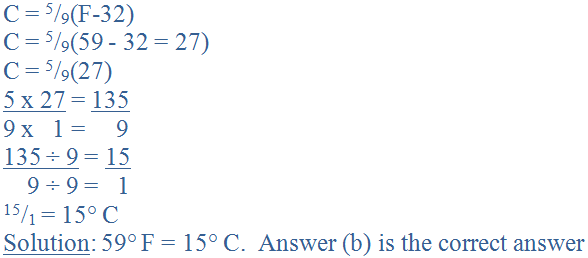Question 6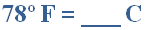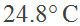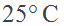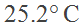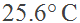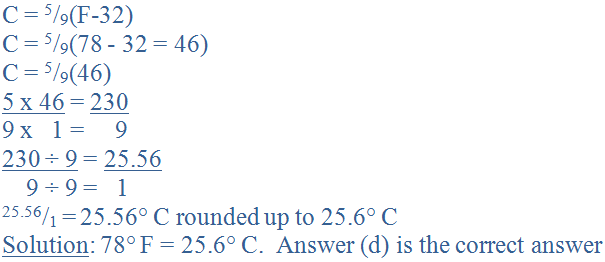Question 7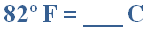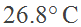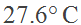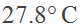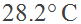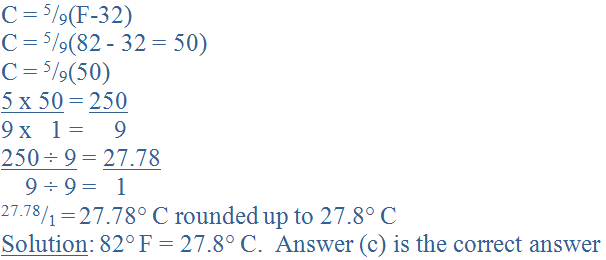Question 8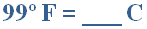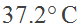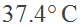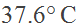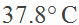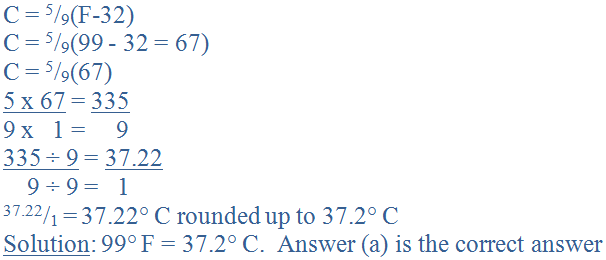Question 9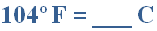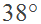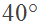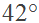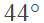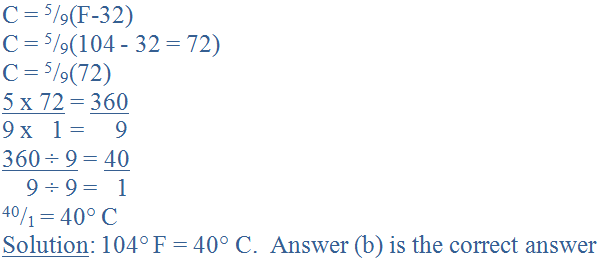Question 10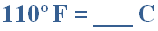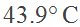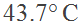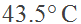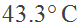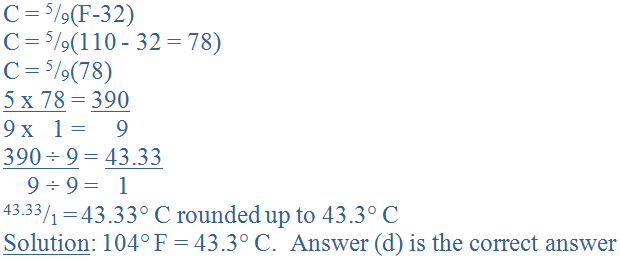Author:  Christine G. Broome